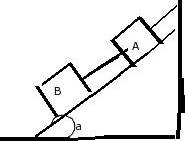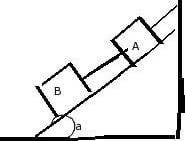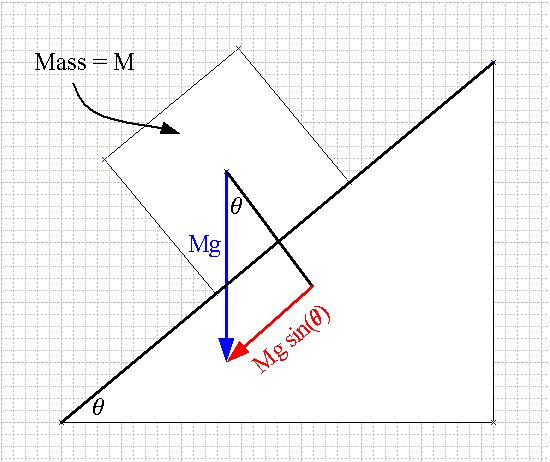# Tension Rope and Blocks Problem

## Homework Statement

Two blocks, each with weight w, are held in place on a frictionless incline as shown in Figure. In terms of w and the angle a of the incline, calculate the tension in the rope connecting the blocksT = mg

## The Attempt at a Solution

I cannot for figure out why the answer is Wsin(a)! I thought that since Tsin(a) = mg, T = w/sin(a)

## Answers and Replies

You have to look at the forces that are pulling along the surface. The force pulling downwards the slope is ##F_d=mgsin(a)##, the force pulling up is just the tension ##T##. For the block to be not moving along the slope these two have to be equal to eachother.

gneill
Mentor

## Homework Statement

Two blocks, each with weight w, are held in place on a frictionless incline as shown in Figure. In terms of w and the angle a of the incline, calculate the tension in the rope connecting the blocksT = mg

## The Attempt at a Solution

I cannot for figure out why the answer is Wsin(a)! I thought that since Tsin(a) = mg, T = w/sin(a)
Hi DaveTan, Welcome to Physics Forums.

Take a close look at the geometry of a block on a slope. The weight W = Mg forms the hypotenuse of the force triangle:Thanks! But shouldn't tension be pointing in the other direction? So towards the top-right instead of bottom left?

haruspex
Science Advisor
Homework Helper
Gold Member
2020 Award
Thanks! But shouldn't tension be pointing in the other direction? So towards the top-right instead of bottom left?
Tension acts both ways (as does compression). The force it exerts on B will be up and to the right, that on A down and to the left.
By the way, the reason your original approach went wrong is that the vertical forces include a component of the normal force.